# Math in Focus Kindergarten Chapter 2 Answer Key Numbers to 10

Go through the Math in Focus Grade K Workbook Answer Key Chapter 2 Numbers to 10 to finish your assignments.

## Math in Focus Kindergarten Chapter 2 Answer Key Numbers to 10

Match.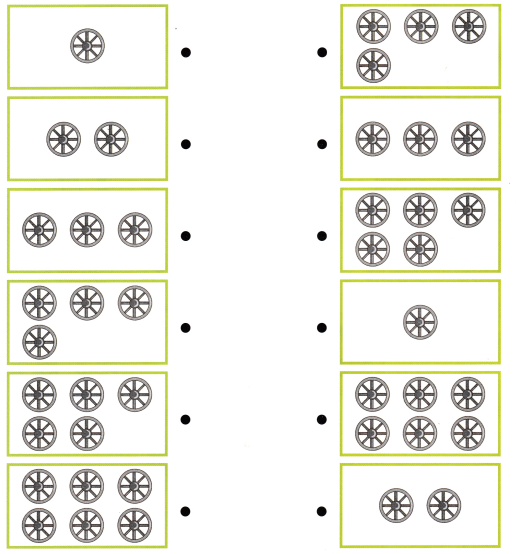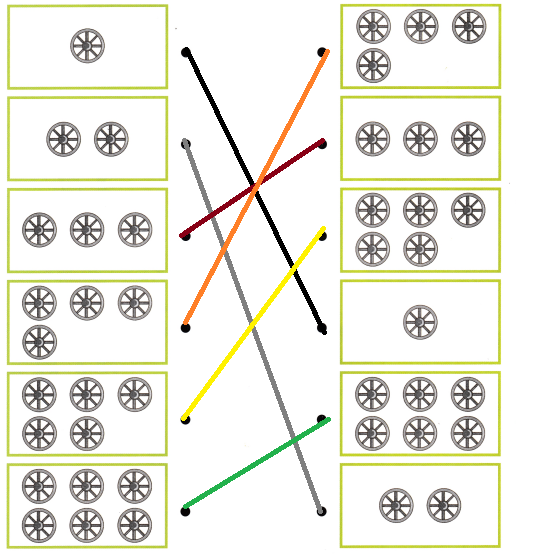Explanation:
Counted the number of wheels and  counted and matched the same number of wheels

Draw.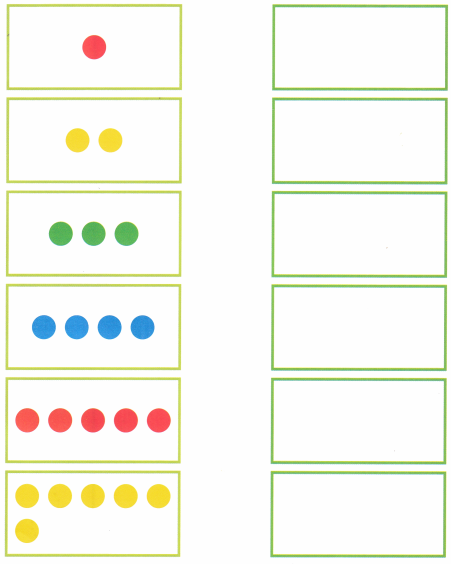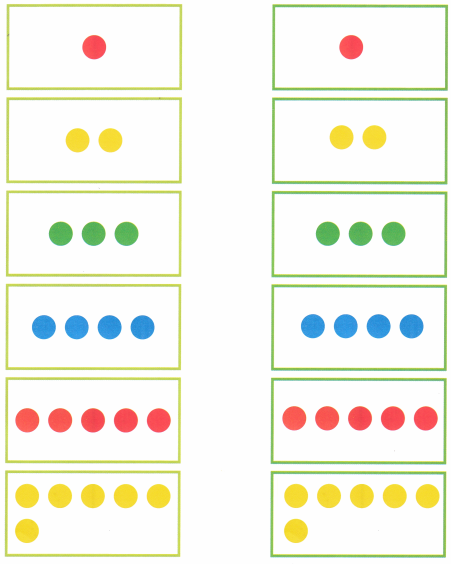Explanation:
counted and  Drawn the number of dots

Trace.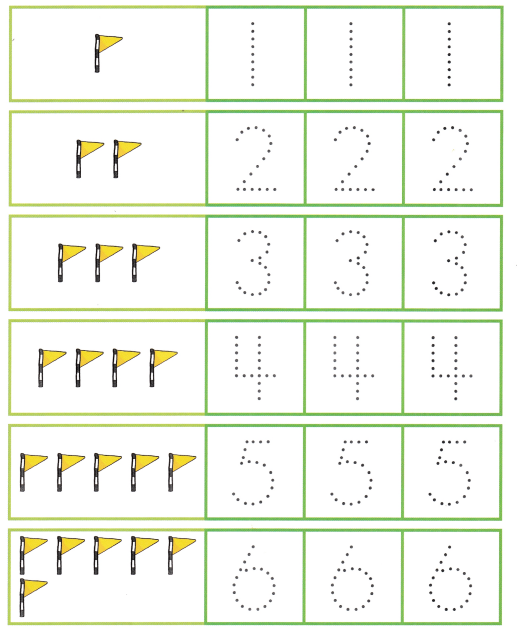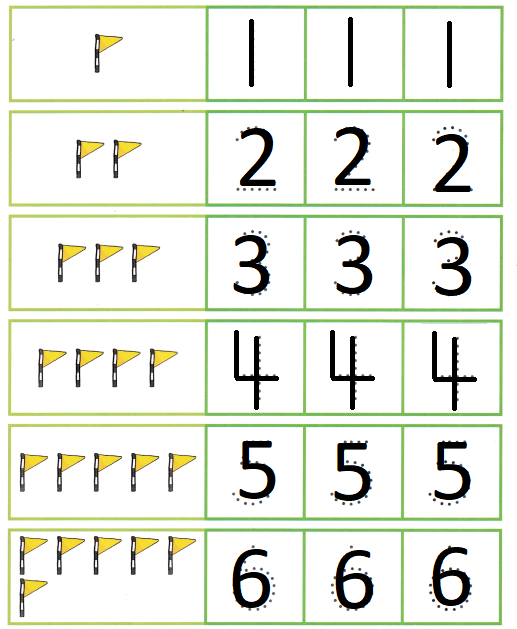Explanation:
Traced the numbers from 1 to 6

Count and Write.

Question 1.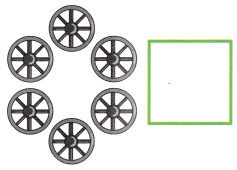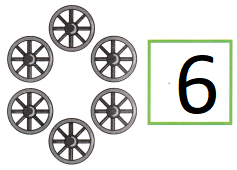Explanation:
Counted and written number of wheels
There are 6 wheels

Question 2.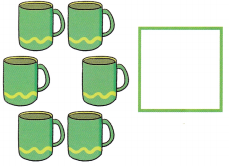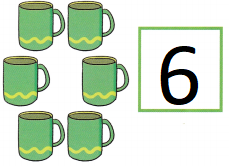Explanation:
Counted and written number of cups
There are 6 cups

Question 3.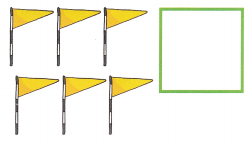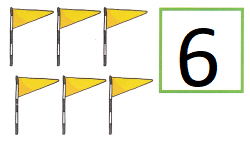Explanation:
Counted and written number of flags
There are 6 flags

Question 4.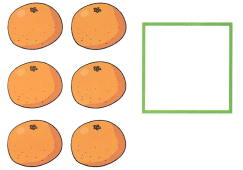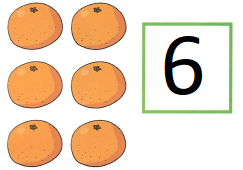Explanation:
Counted and written number of oranges
There are 6 oranges

Question 5.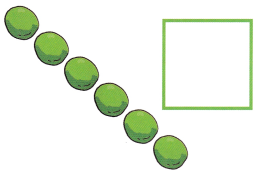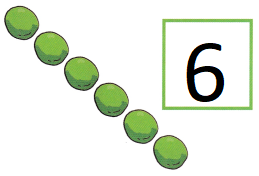Explanation:
Counted and written number of lemons
There are 6 lemons

Question 6.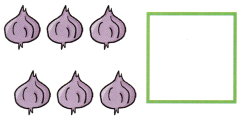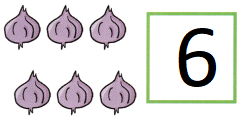Explanation:
Counted and written number of onions
There are 6 onions

Sing.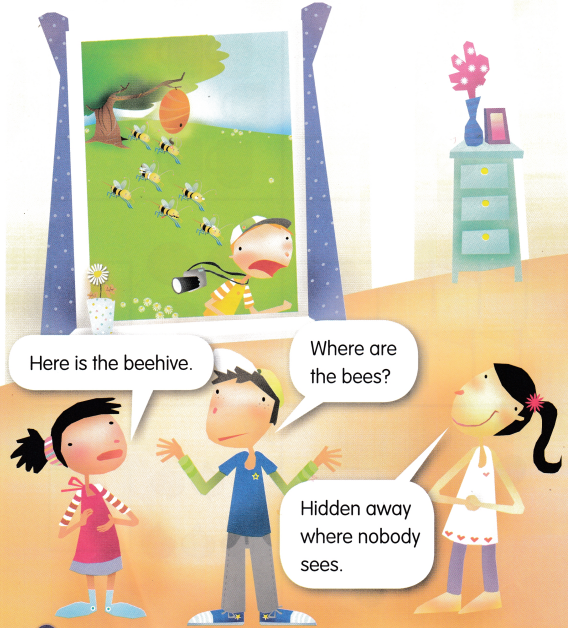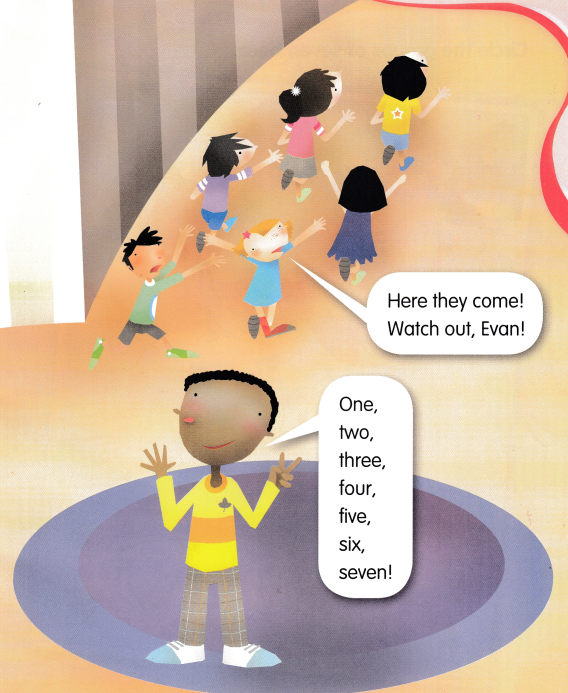Circle the groups of seven bees.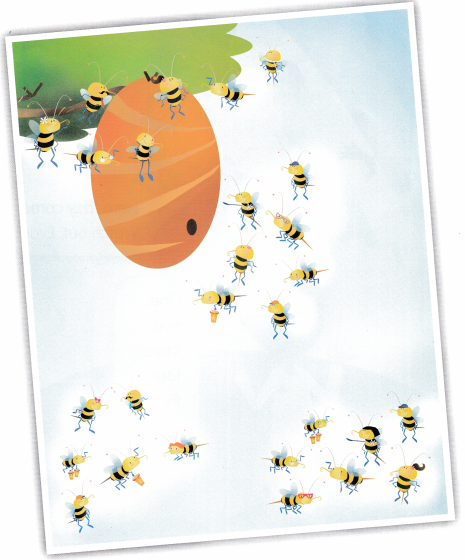Explanation: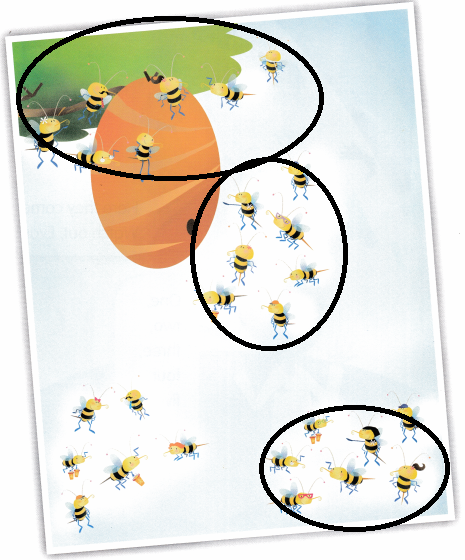Circled the group of seven bees

Match.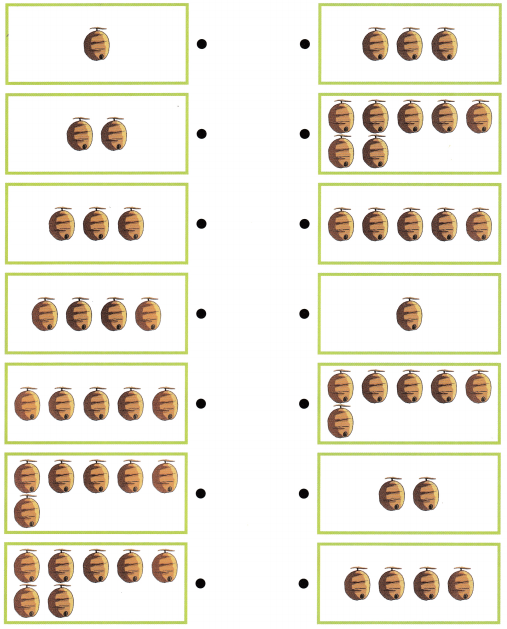Explanation: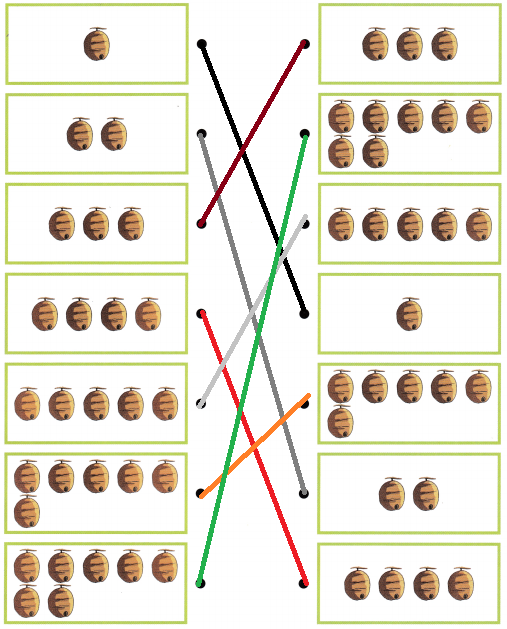Counted the number of hives and matched with the same number of hives

Draw.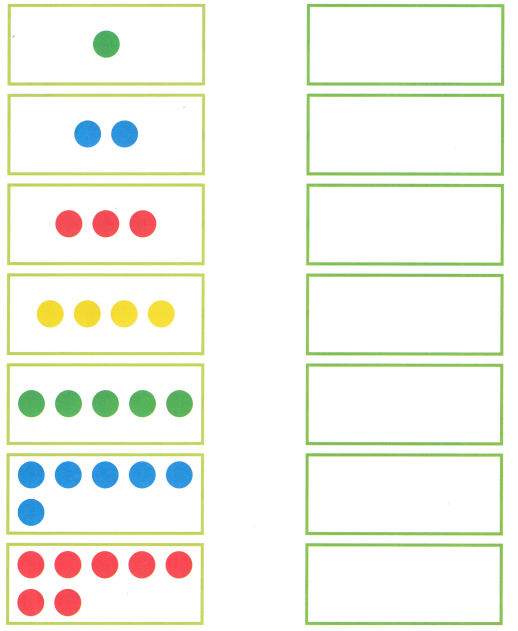Explanation: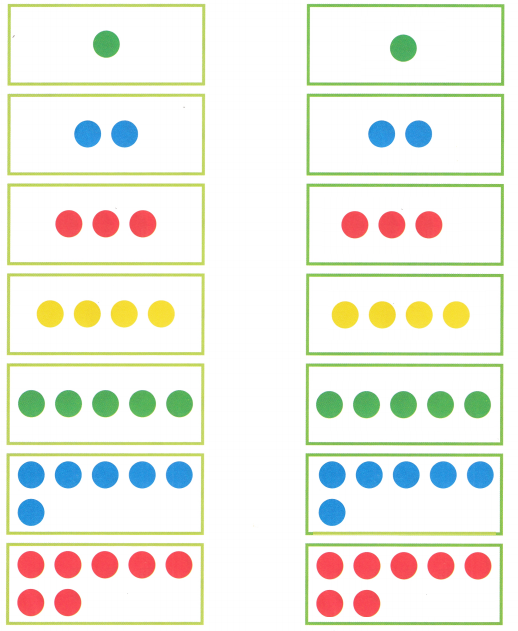Counted and drawn the circles

Trace.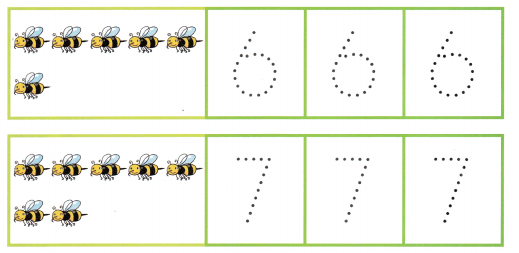Explanation: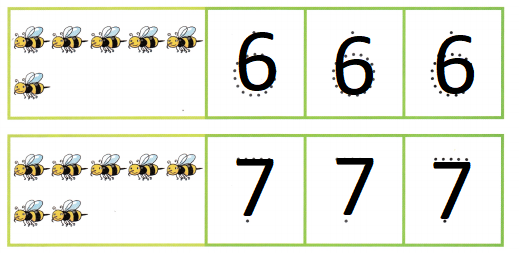Traced the numbers from 6 and 7

Look and Say.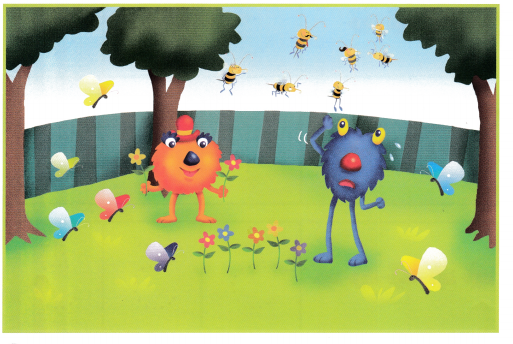Count and Write.

Question 1.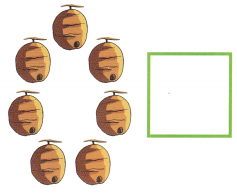Explanation:
Counted and written number of hives
There are 7 hives

Question 2.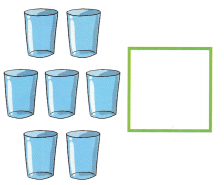Explanation:
Counted and written number of glasses
There are 7 glasses

Question 3.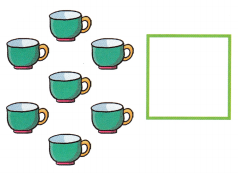Explanation:
Counted and written number of cups
There are 7 cups

Question 4.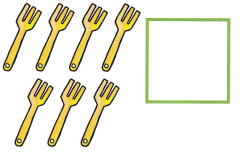Explanation:
Counted and written number of forks
There are 7 forks

Question 5.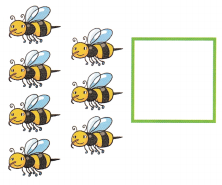Explanation:
Counted and written number of bees
There are 7 bees

Question 6.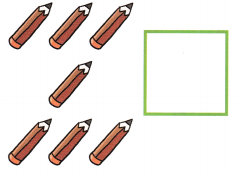Explanation:
Counted and written number of pencils
There are 7 pencils

Color.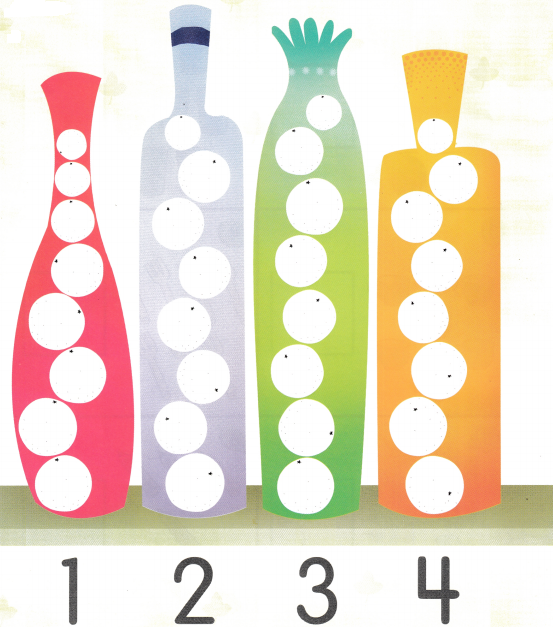Explanation: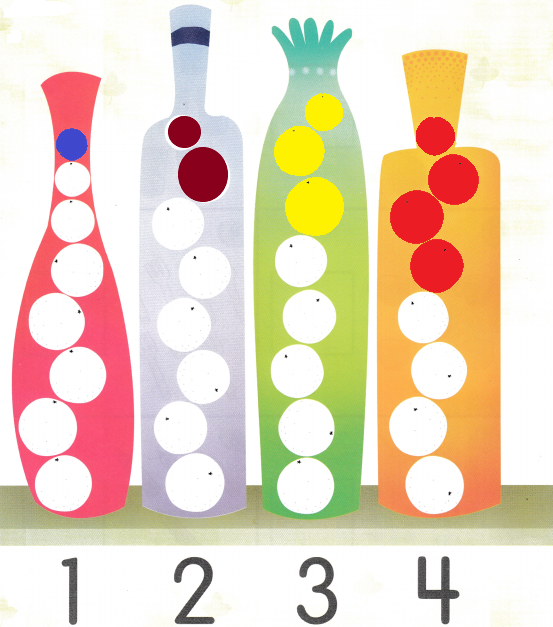According to the number colored the dots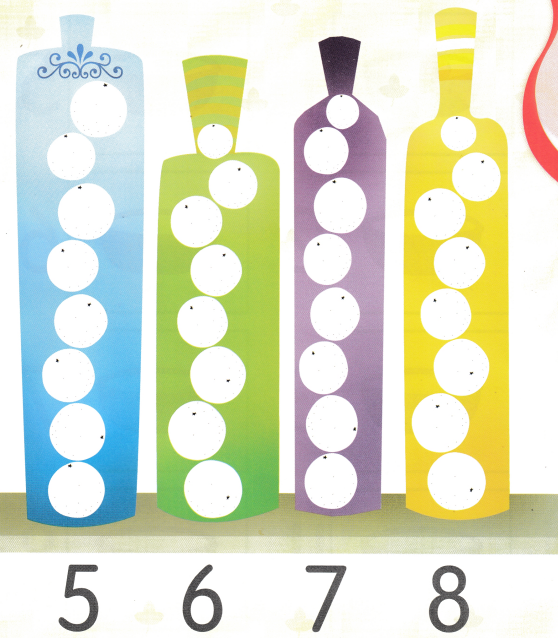Explanation: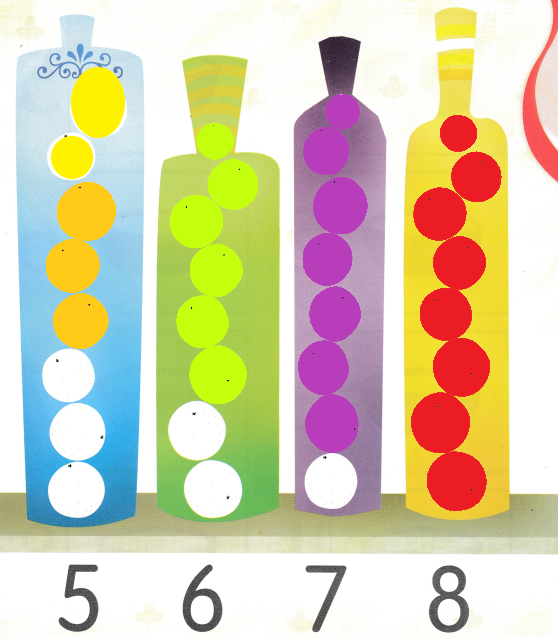According to the number colored the dots

Draw.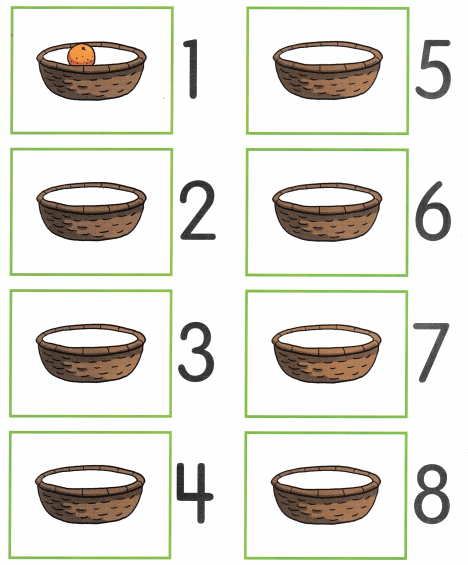Explanation: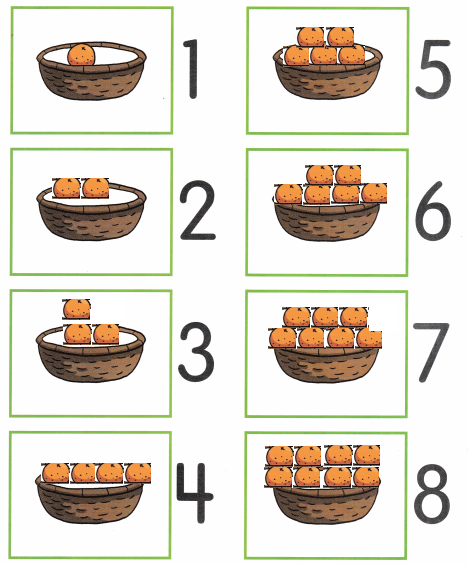According to the numbers drawn the apples

Match.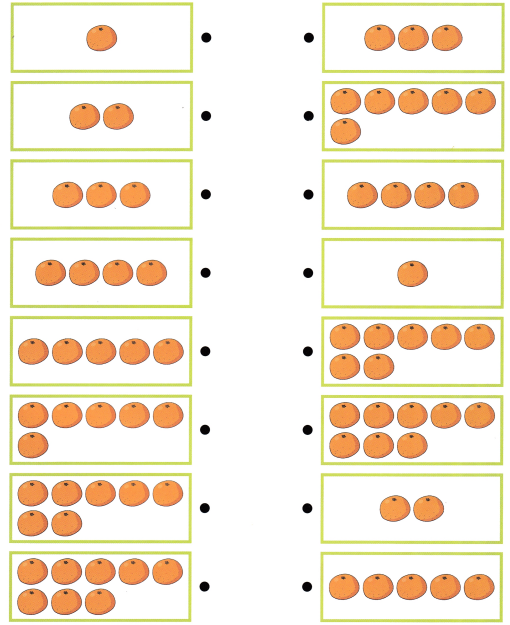Explanation:
Counted the number of oranges and matched according to the same number of oranges

Draw.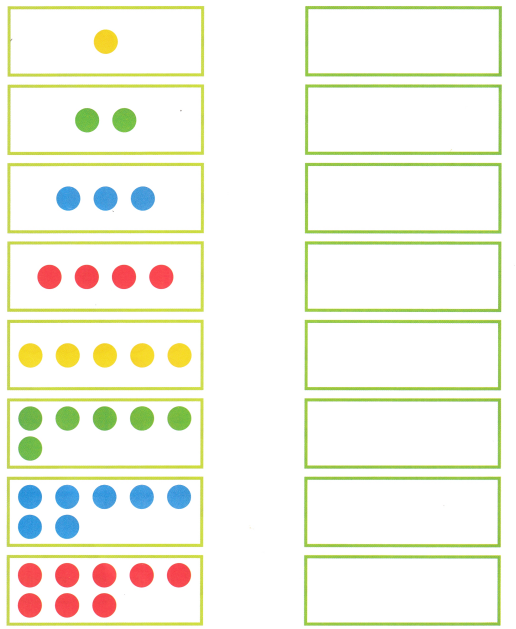Explanation: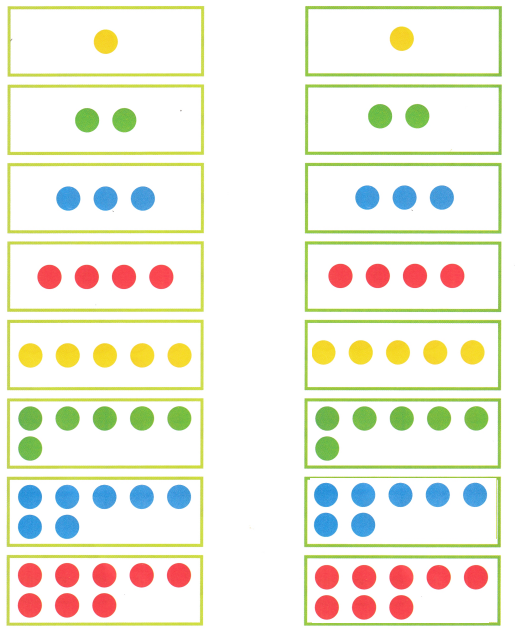Counted and drawn the same number of dots

Trace.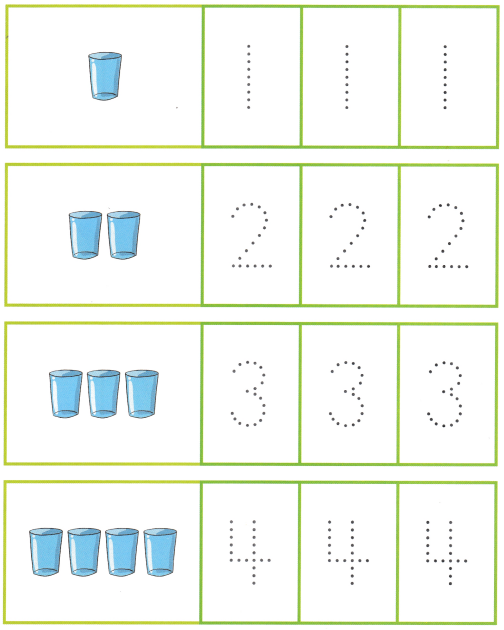Explanation: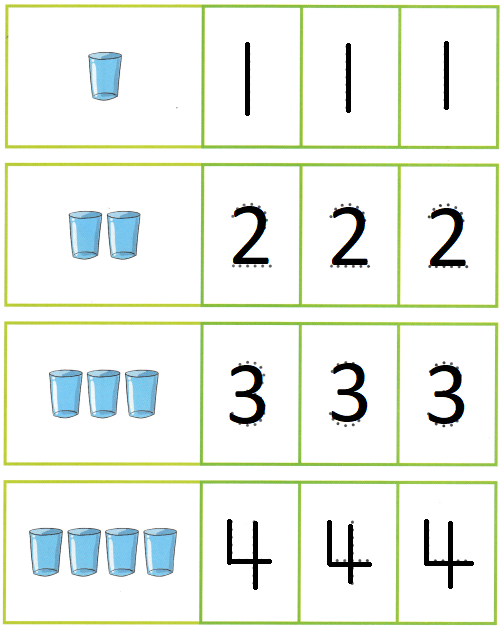Written and traced the numbers from 1 to 4

Trace.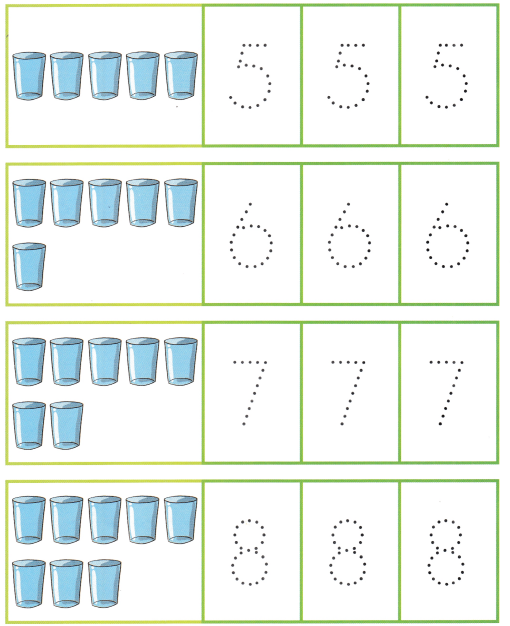Explanation: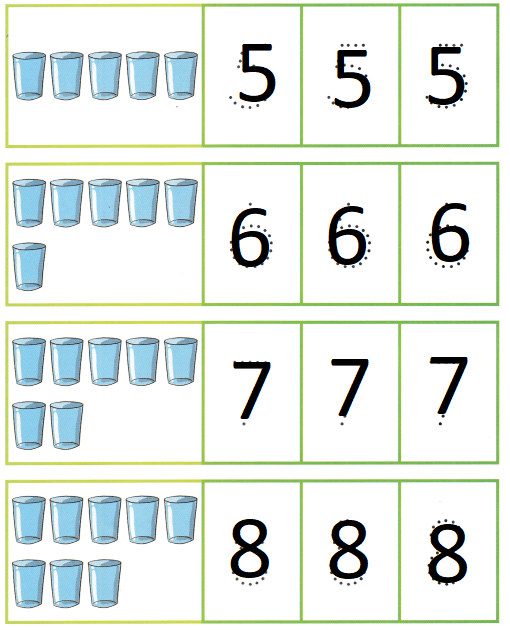Written and traced the numbers from 5 to 8

Count and write.

Question 1.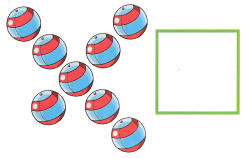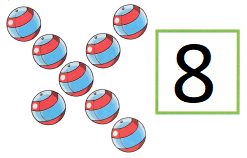Explanation:
Counted and written number of balls
There are 8 balls

Question 2.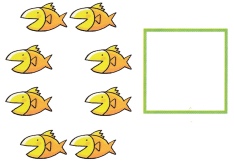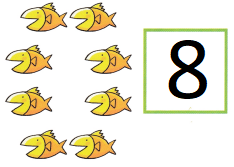Explanation:
Counted and written number of fishes
There are 8 fishes

Question 3.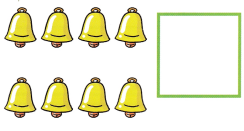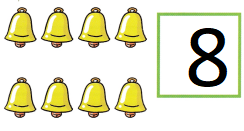Explanation:
Counted and written number of bells
There are 8 bells

Question 4.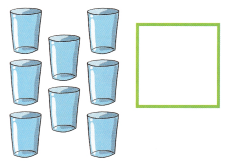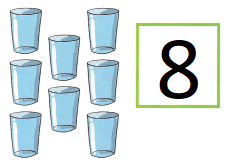Explanation:
Counted and written number of glasses
There are 8 glasses

Question 5.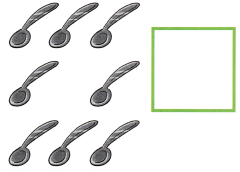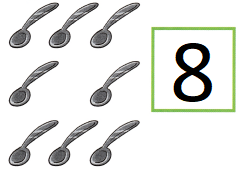Explanation:
Counted and written number of spoons
There are 8 spoons

Question 6.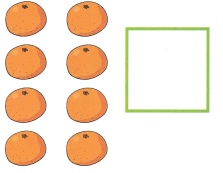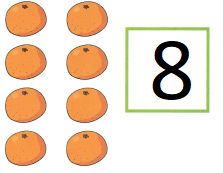Explanation:
Counted and written number of oranges
There are 8 oranges

Lesson 4 Numbers 0 to 9

Match.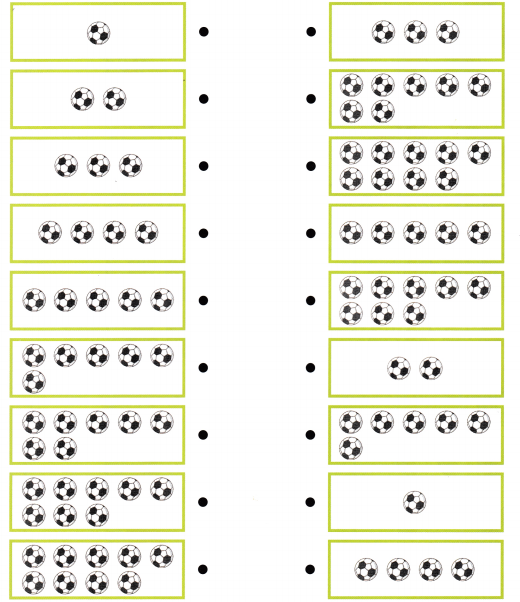Explanation: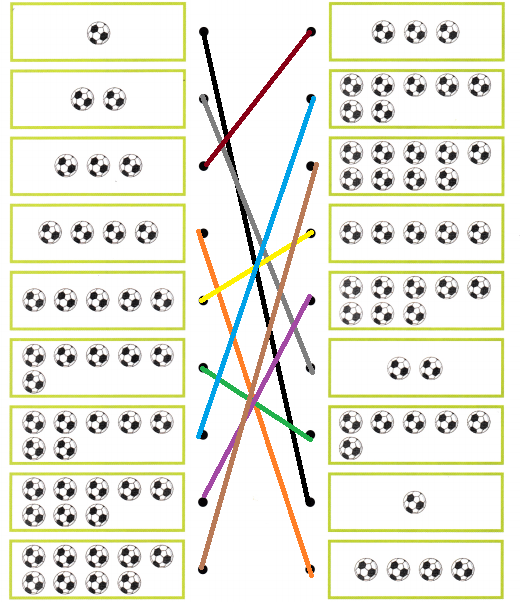Counted and matched the numbers from 1 to 9

Draw.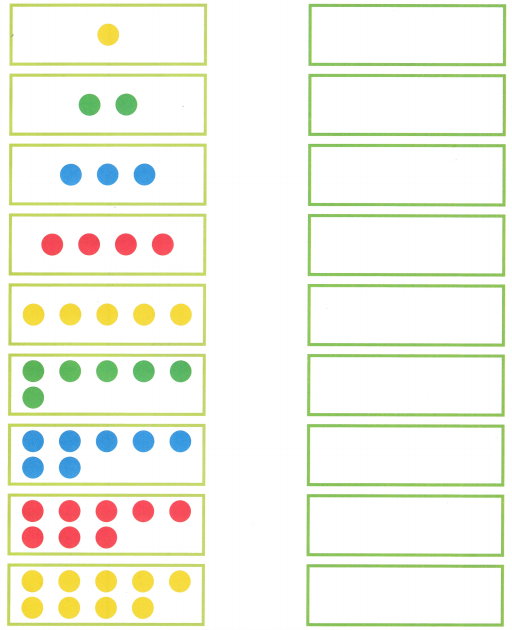Explanation: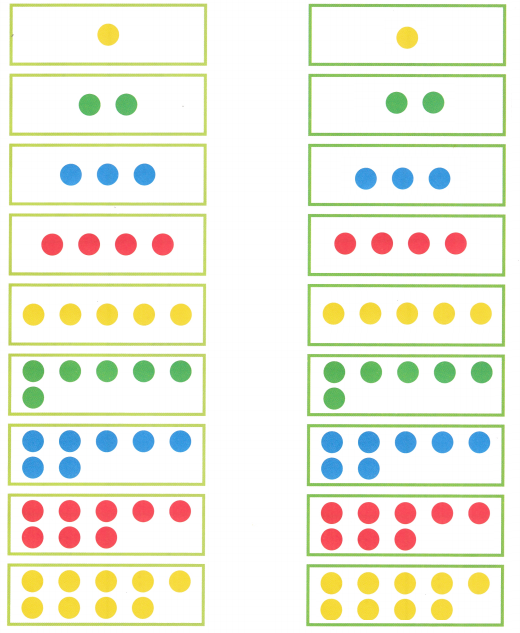Drawn the same number of dots by counting the dots

Trace.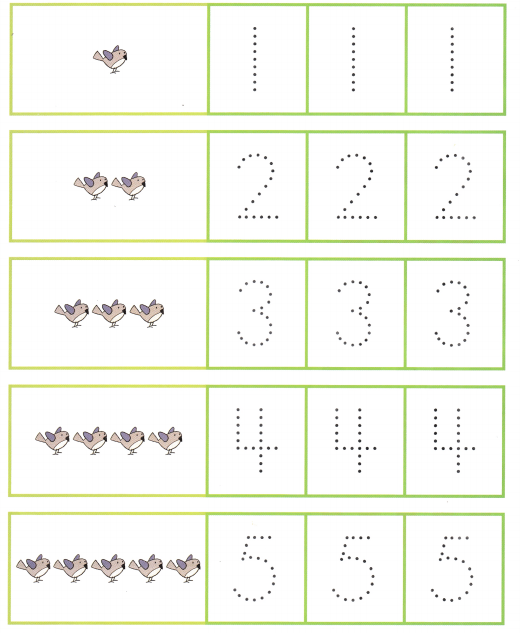Explanation: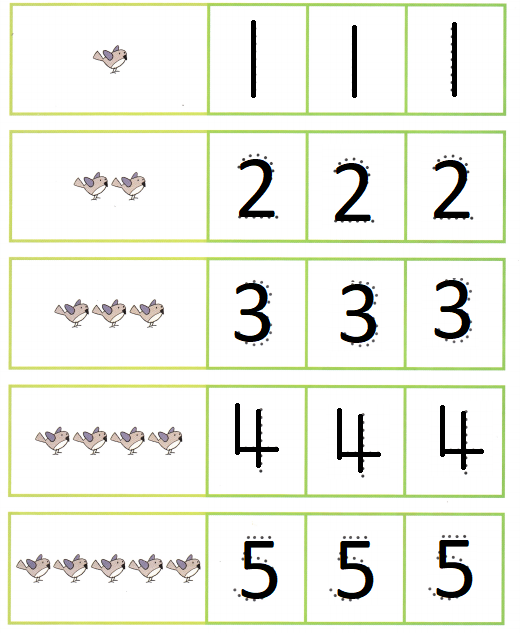Traced the numbers from 1 to 5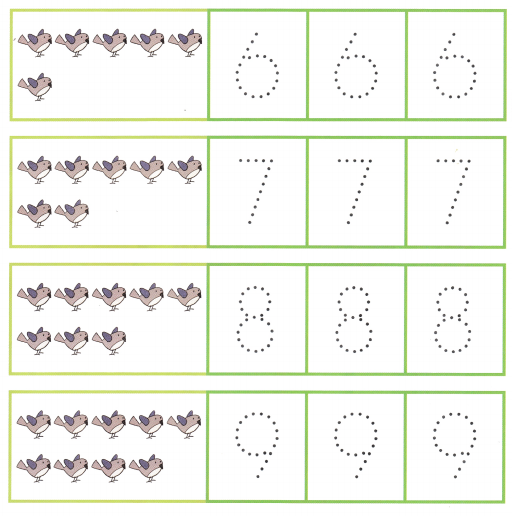Explanation: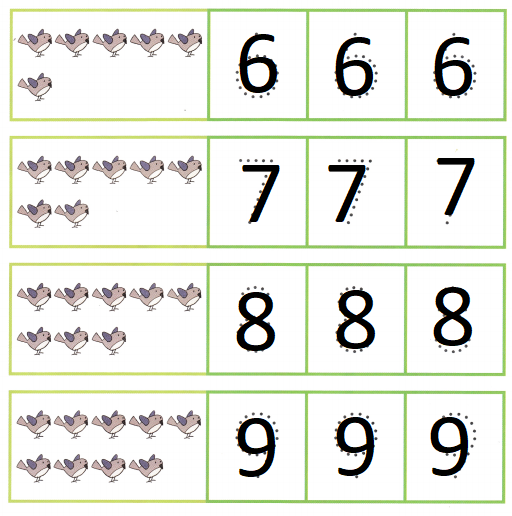Traced the numbers from 6 to 9

Write the missing numbers.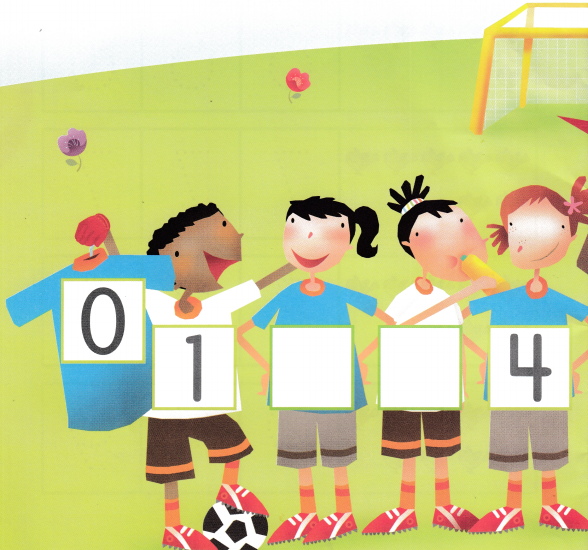Explanation: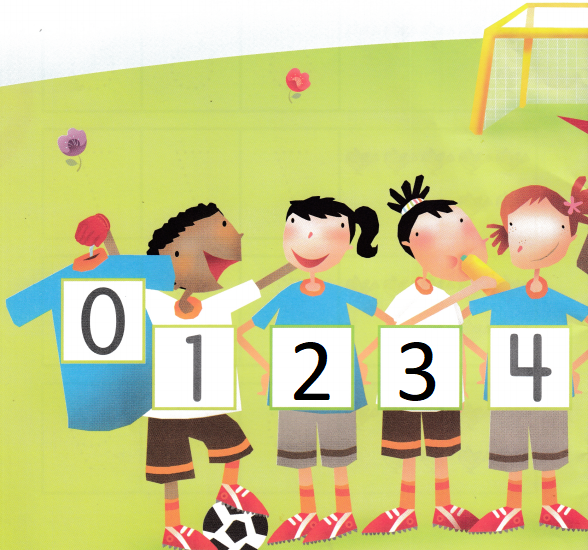Written the missing numbers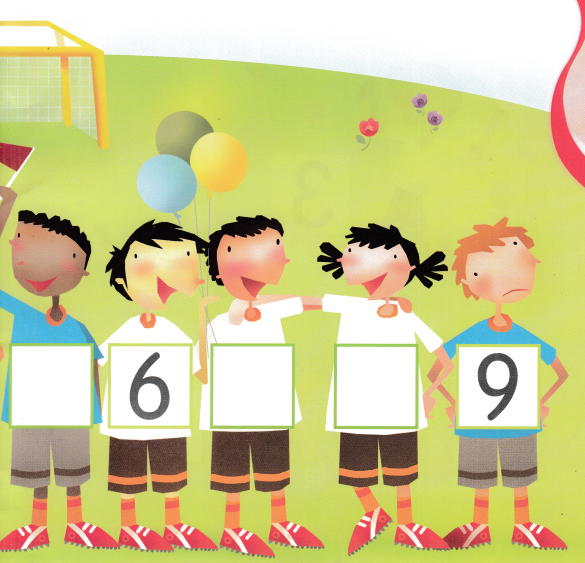Explanation: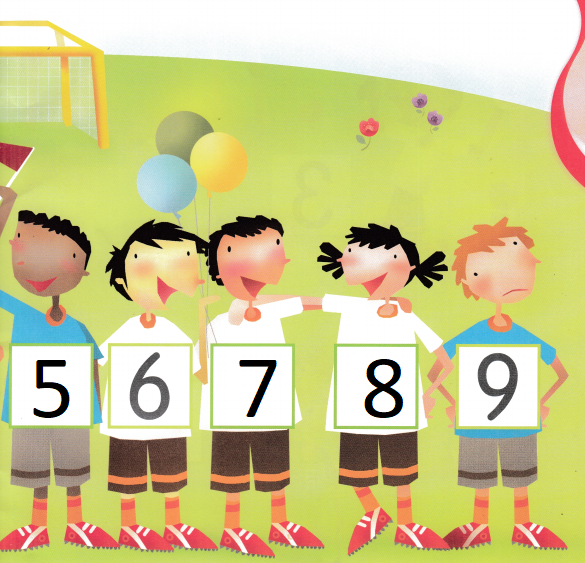Written the missing numbers

Count and write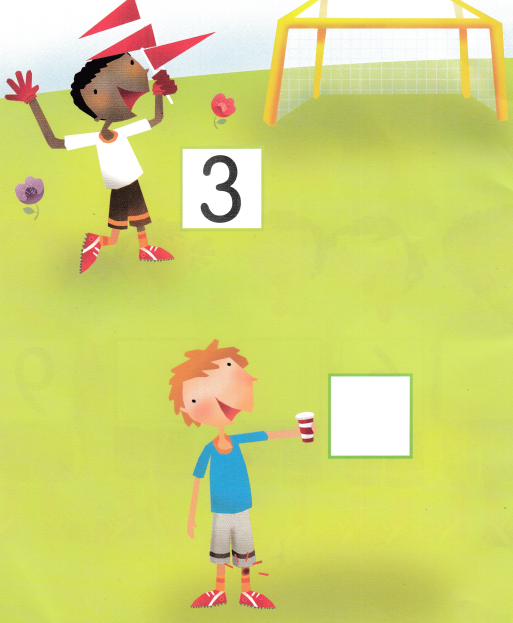Explanation: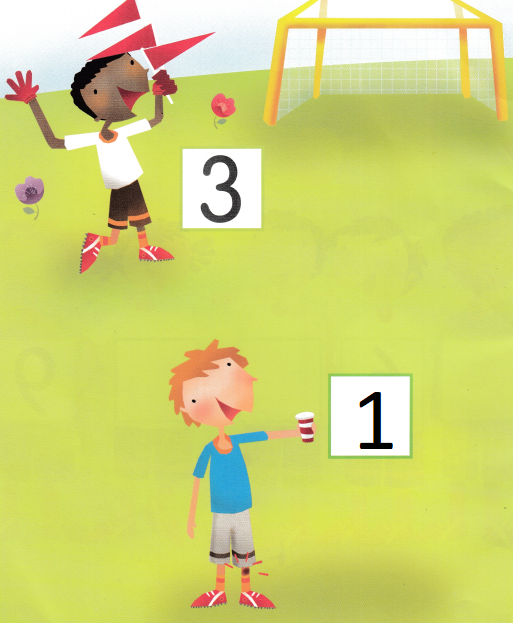The second boy is holding only one glass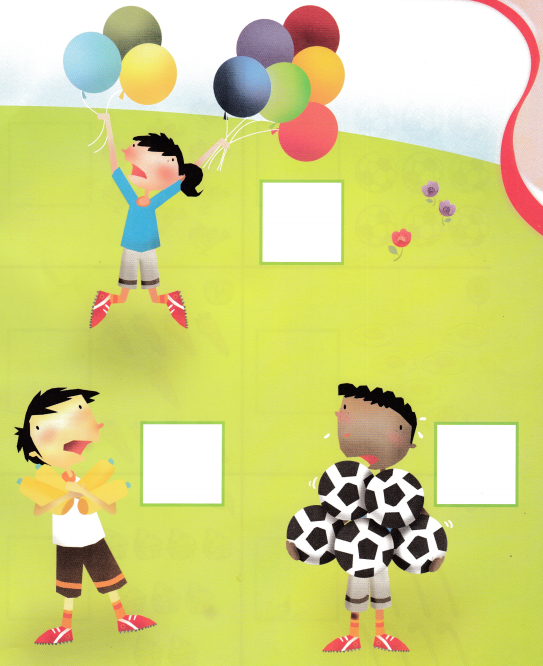Explanation: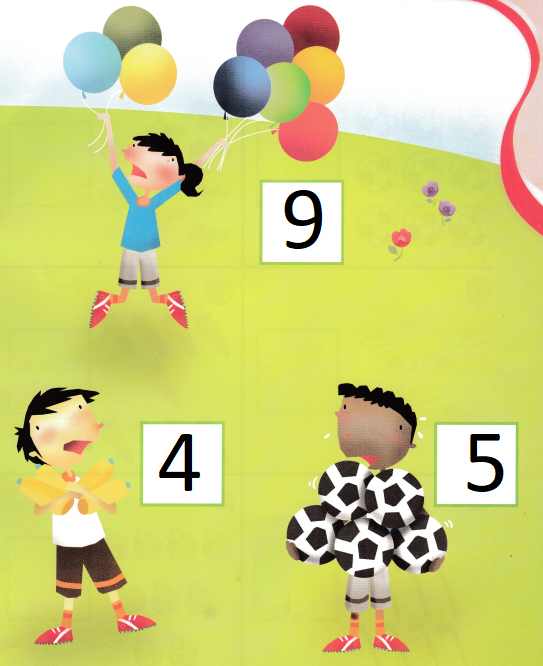In the above picture the first boy is catching 9 balloons
the second boy is catching 4 bottles and the third boy is catching 5 balls

Count and write.

Question 1.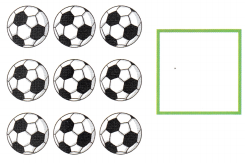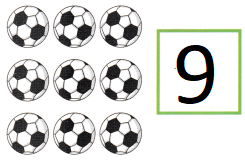Explanation:
Counted and written number of Balls
There are 9 balls

Question 2.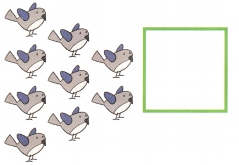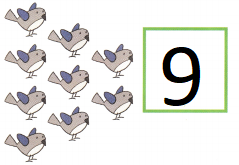Explanation:
Counted and written number of birds
There are 9 birds

Question 3.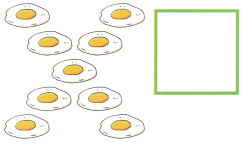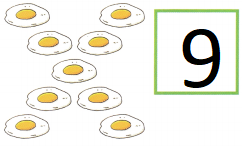Explanation:
Counted and written number of Eggs
There are 9 eggs

Question 4.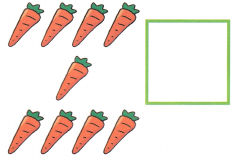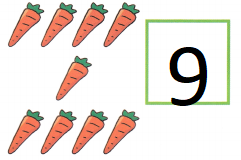Explanation:
Counted and written number of Carrots
There are 9 carrots

Question 5.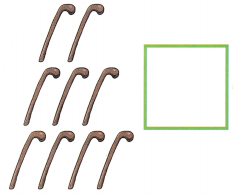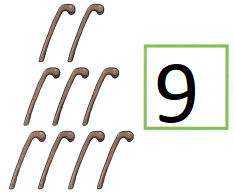Explanation:
Counted and written number of sticks
There are 9 sticks

Question 6.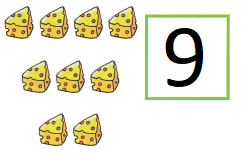Explanation:
Counted and written number of Cheese
There are 9 cheese

Circle the group of 9 players.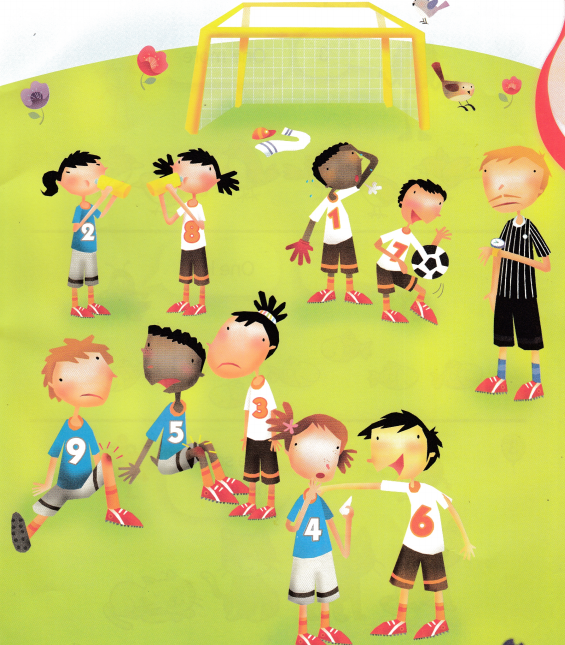Explanation:
In the above picture there are 10 boys circled 9 of them

Lesson 5 Pairing Sets with Numbers

Question 1.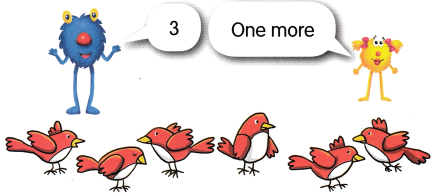Explanation:
3 + 1 = 4
Circled the 4 birds

Question 2.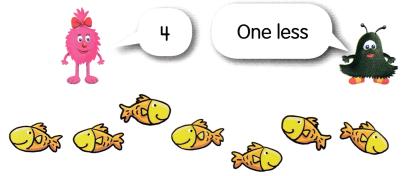Explanation:
4 – 1 = 3
Circled 3 fishes

Question 3.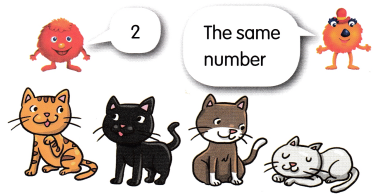Explanation:
Circled the same number that is 2

Lesson 6 Pairing One-to-One

What is missing? Complete the set.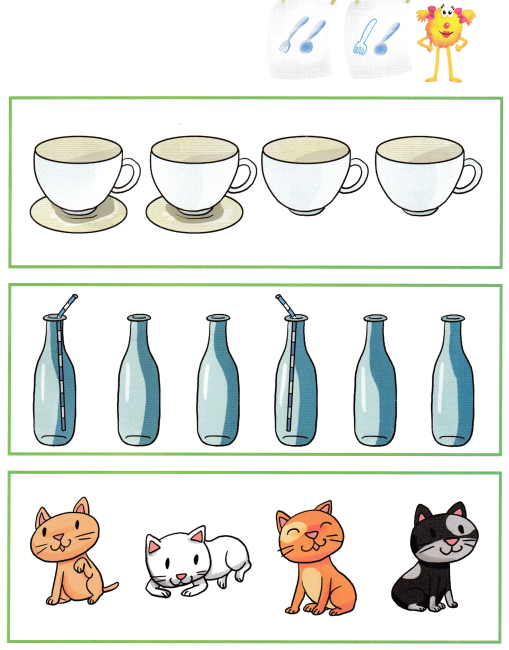Explanation:
For cups saucer is missing
For bottles straw is missing
For cats tails are missing

Circle, Count, and write.

Question 1.
There is cheese for 6 mice.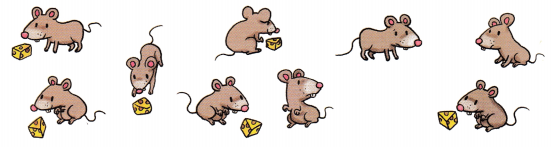How many mice will be hungry? ____
3 will be hungry
Explanation:
There are 9 mice but only 6 cheese are there so 3 are missing

Question 2.
3 boys have coats.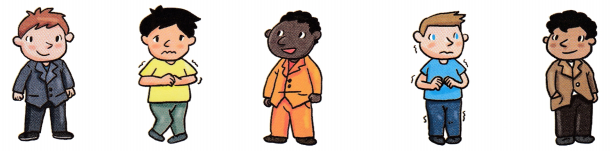How many boys will be cold? ____
2 boys will be cold
Explanation:
There are 5 boys out of them 3 having coats
so 2 will be cold

Question 3.
There are 6 egg holders.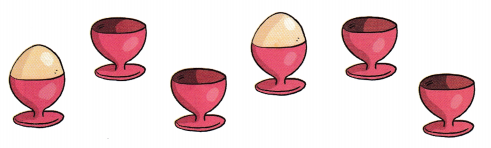How many eggs are needed to fill all the egg holders? _____